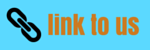# Gravitation

## Free Fall

• Due to gravitational force Earth attracts every object towards it.
• So whenever an object falls towards earth it falls under the influence of gravitational force and if only gravitational force acts on the object during its free fall then such a motion of object is said to be free fall motion
The falling of a body (or object) from a height towards the earth under the gravitational force of earth (with on other force acting on it) is called free fall
• When an object falls freely towards the earth there happens to be no change in the direction of motion of the object but due to the attraction of earth there will be a change in the magnitude of velocity.
• Change in velocity involves acceleration. So whenever the objects falls towards earth an acceleration is involved due to earth's gravitational force.
• This acceleration due to earth's gravitational force is called acceleration due to gravity which is denoted by 'g' and its unit is m/s2

### Calculation of value of g

• We shall now find the acceleration due to gravity 'g'
• Let us consider an object of mass $m$ falling freely under the influence of Earth's gravitational force.
• Since the object is moving under the sole effect of gravity (free fall motion) then acceleration experienced by the object would be g which is the acceleration due to gravity of earth.
• Now from Newton's second law of motion , force experienced by this freely falling object would be
$F_{grav}=mg$                 (5)
• Again from Universal law of gravitation force of gravity with which an object is attracted to earth is
${F_{grav}} = G\frac{{{M_{earth}} \times m}}{{{d^2}}}$              (6)
where $d$ is the distance from the center of the object to the center of the earth and $M_{earth}$ is the mass of the earth.
• Now from equations (5) and(6) we have $mg = G\frac{{{M_{earth}} \times m}}{{{d^2}}}$
or, $g = G\frac{{{M_{earth}}}}{{{d^2}}}$                (7)
• So if the object under consideration is very near to the surface of the earth or on the surface of the earth then we can approximate $d \approx {R_{earth}}$ where $R_{earth}$ is the radius of the earth. So expression for g near or on the surface of the earth would be
$g = G\frac{{{M_{earth}}}}{{{{({R_{earth}})}^2}}}$                (8)
• One more important point here to note is thatearth is not a perfect sphere. As the radius of earth increases from the poles to the equator , the value of g becomes greater at the poles then at the equator
• Now to calculate the value of g we shall put the values $G = 6.7 \times {10^{ - 11}}N{m^2}K{g^{ - 2}}$
${R_{earth}} = 6.4 \times {10^6}m$
${M_{earth}} = 6 \times {10^{24}}Kg$
So,
$g = G\frac{{{M_{earth}}}}{{{{({R_{earth}})}^2}}}$
putting in above values and calculating we find
$g = 9.8m{s^{ - 2}}$

### motion of objects under the influence of Earth's gravity

• From equation (8) we can see that the acceleration that object experiences during the free fall is independent of the mass of the object undergoing motion
• This implies that both light or heavy, small oor big, hollow or solid objects would fall at the same rate.
• As the value of acceleration due to gravity is constant near earth , we can now write the equations of motion that we studied in chapter motion would be valid for free fall motion if we replace acceleration 'a' with acceleration due to gravity 'g'
• So in this case equations of motion would be
$v=u+gt$     when object is falling downwards
$v=u-gt$     when object is thrown upwards
$h=u+\frac{1}{2}gt^{2}$
abd $v^{2}-u^{2}=2gh$
where $h$ is the height of the object from the ground.